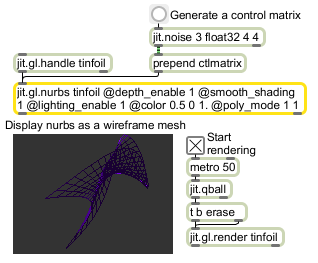jit.gl.nurbs

Generate NURBS surface

Description

The jit.gl.nurbs object renders a Non-Uniform Rational B-Spline (NURBS) surface. A NURBS is a mathematical model that lets you represent virtually any desired shape, from points, straight lines, and polylines to conic sections (circles, ellipses, parabolas, and hyperbolas) to free-form curves with arbitrary shapes. You can also control the shape of a curve a NURBS generates via sets of control points and knots that can be altered to control smoothness and curvature. A NURBS surface is also an economical way to represent complex shapes with very little data.

A control matrix may specify the control points. The order of the curve may be specified for both the x and y axes (which are sometimes referred to as the u and v surface axes). The order of the curve must be less than the number of control points across the associated dimension. The default order is 3 (i.e., cubic).

At present, the knots sequence is automatically generated based a sum equal to the curve + number of control points. Control points may be non uniformly weighted if the control matrix has 4 planes (x, y, z, w) where w is the weight value. By default, the control matrix is a random 4x4 matrix of uniformly weighted points.

The jit.gl.nurbs object requires one argument: the name of a drawing context. A named drawing context is a named instance of a jit.window , jit.pwindow , or jit.matrix object that has an instance of the jit.gl.render object associated with it. This value may also be set via the OB3D drawto attribute.

Messages

 ctlmatrix matrix-name [symbol] Copies the named matrix to the control point matrix. Matrices must have a planecount of 3 or 4, and should be typefloat32 or float64 rand Generates a random set of control points.

Attributes

Name Type g/s Description
closed int The closed flag for each axis inf the form x y (default = 0 0). If closed about an axis, the nurbs surface will knot to smoothly close the surface across that axis.
ctlshow int Control point rendering flag (default = 0) When the flag is set, the control points are rendered.
dim int The dimensions of the grid (default = 20 20)
displaylist int Cache in displaylist flag (default = 0) This feature may be used to speed up rendering time by creating and storing a list of gl drawing commands on the graphics card. This will have no effect if matrixoutput turned on.
order int The interpolation order in the form x y (default = 3 3). The minimum interpolation order is 1 1. The maximum order is set by the values (control; max = control matrix width-1 height-1)

ExamplesName Description
jit.gl.graph Open GL floating-point data visualization
jit.gl.gridshape Generate simple geometric shapes as a connected grid
jit.gl.handle Use mouse movement to control position/rotation
jit.gl.isosurf Generates a GL based surface extraction
jit.gl.mesh Generates GL geometry from existing data
jit.gl.model Read and draw Wavefront .obj models
jit.gl.plato Generate platonic solids
jit.gl.render Render Open GL